Chronology of the KAM Theorem and the Outer Billiards Map ●1892: Poincare  J.H. Les methodes nouvilles de la mechanique celeste,  Paris: Gauthier-Villars In 1887, in honor of the 60th birthday of King Oscar II of Sweden, a prize was offered to anyone who could solve the following problem, due to Karl Weierstrauss:   "Given a system of arbitrary mass points that attract each other according to Newton’s laws, under the assumption that no two points ever collide, try to find a representation of the coordinates of each point as a series in a variable that is some known function of time and for all of whose values the series converges uniformly."   This was the famous n-body problem which had its beginnings in the work of Isaac Newton (1642-1727) for the Sun, Earth and Moon. But this 'lunar' problem was not trivial and the general n-body solution, taking into account the gravitational interaction of the planets, had proven to be very difficult. In 1710 Johann Bernoulli solved the problem for 2 bodies but his methods did not generalize to 3 or more bodies. At age 35, Henri Poincare submitted the prize-winning paper which showed that there was no way to solve the problem by traditional methods of reducing the dimension using 'integrals' of motion so there were no integral-based 'closed form' solutions. By contrast the 2-body problem is 'integrable'.   The key issue was resonances and 'small divisors' which could lead to unstable (homoclinic) orbits. (This left open the possibility that the n-body problem could be solved by other means, and indeed the 3-body was solved by Karl Sundman in 1906 and the n-body version was solved by Quidong Wang in 1991, but the infinite series used in their solutions converged so slowly that they were of no practical value. And this created a debate among mathematicians about the definition of a 'solution')   Poincare's paper was later expanded to the three volumes of Les methodes nouvilles de la mechanique celeste and this laid the foundation for new areas of study based on qualitative methods. This spelled the end of the 'clockwork universe' of Newton and Leibniz. Together with G. D. Birkoff, Poincare laid the groundwork for topology and the study of manifolds. In his (revised) paper for the prize, Poincare realized that even for 3 bodies there could be solutions which had 'sensitive dependence on initial conditions' and this would make prediction impossible. This condition is now the key ingredient of chaos theory. Below is a chaotic orbit for the 3-body problem.●1942: Siegel C.L.  Iteration of (Complex) Analytic Functions, Ann of Math  42: 607-612 The real issue for the n-body problem is whether there are 'stable' solutions. Poincare was of the opinion that 'most' solutions would be unstable because of the small divisor problem. The small divisor problem has its source in irrationally indifferent fixed points.These are 'elliptic' fixed points which are neither attracting or repelling and where the corresponding linear map is an irrational rotation θ. In complex analysis these points are know as holomorphic germs. Poincare stated the problem as follows:   Given a holomorphic (complex analytic) function such as f(z) = ρz + z2, with ρ = ei2πθ, for what values of θ will f  be linearizible near the fixed point z = 0 ?                                                For such fixed points it is not clear whether the system will be stable under small perturbations, because nearby rational rotations lead to small divisors and are typically not stable. In 1942 Carl Siegel showed that for complex analytic functions, the small divisor problem can be overcome for these fixed points if θ satisfies a Diophantine condition which guarantees it cannot be approximated closely by rationals. This showed that, from the standpoint of complex analysis, the small divisor problem was a matter of degree, so some solutions will converge and others will diverge.   In Paris, Gaston Julia (1893-1978) and Pierre Fatou (1878-1929) were also interested in this problem. They were trying to classify fixed points of complex rational functions based on stability and the irrationally indifferent case was the most difficult. Fatou thought that such points were never stable because of the small divisor problem. On the left below is the Julia set for an irrationally indifferent fixed point and on the right is the Jilia set for a rationally indifferent fixed point. In this case, the chaotic dynamics reach all the way to the fixed point so there is no stable neighborhood, while on the left the fixed point is surrounded by invariant rings which testify to the local stability.This stable region is known as a Siegel Disk. The boundary of this disk is typically very complex.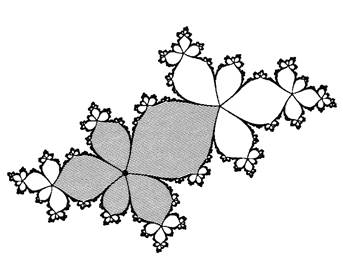●1954: Kolmogorov  A.N.  Preservation of Conditionally Periodic Movements with Small Change in the Hamiltonian Function,  Doklady Academii Nauk (Report of the Academy of Sciences) SSSR vol. 98 : 527-530. In the early 1950's A.N.Kolmogorov was the most influential mathematician in Russia, but his work not widely known to the outside world because of the Iron Curtain. In an 1954 address to the International Congress of Mathematicians he laid out a plan to resolve Poincare's stability problem using new iterative convergence methods. Starting with an integrable Hamiltonian system, the issue is whether the system can remain stable under small perturbations. Jurgen Moser, wrote a review of Kolmogorov's proposal and pointed out that there were still some important unresolved issues. Moser felt that there was promise in Kolmogorov's ideas and he set out to resolve these issues on his own. Even though Moser was a friend and colleague of Carl Siegel, he did not base his work on complex analytic functions because these were too 'well behaved' to apply to a wide class of Hamiltonian (conservative) systems. He chose to assume a 'smooth' real-valued function and meanwhile a brilliant young protégé of Kolmororov named V.I. Arnold was developing a proof based on real analytic Hamiltonian functions.●1962: Moser J.K. On invariant curves of area-preserving mappings of an annulus, Nachr. Akads. Wiss, Gottingen, Math. Phys.   The main result is called the Twist Theorem and it addresses the 'center problem' of Poincare, Siegel and Kolmogorov. The critical case occurs when the center is an 'elliptical' fixed point of an integrable Hamiltonian system. The question is whether this fixed point can remain stable under periodic perturbations. To simulate this, Moser used a mapping of the annulus of the form T(ρ,φ) = (ρ,  φ + 2πω). For a fixed radius ρ, the amount of 'twist', ω , is called the ‘winding number’.  It is assumed to be a smooth function of ρ. For fixed ρ, T(φ)= φ + 2πω so points would rotate by 2πω and the orbits can be classified as rational or irrational. The irrational rotations are called quasi-periodic and they are dense in the circle as shown below. The rational rotations yield discrete orbits.   Under periodic perturbations, the rational orbits may break down and form rings of unstable fixed points and stable 'islands' as shown in the middle. This break down is caused by resonances between the orbit and the perturbation. Each of these new fixed points feels the same perturbations so they behave in a similar fashion, creating endless chains of fixed points and 'islands'. If enough of the quasi-periodic orbits survive, this instability is localized. Moser showed that that under certain 'smoothness' conditions on the Hamiltonian function, a finite measure of these invariant quasi-periodic curves will survive the perturbation and guarantee long-term stability.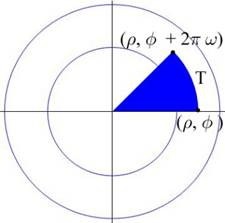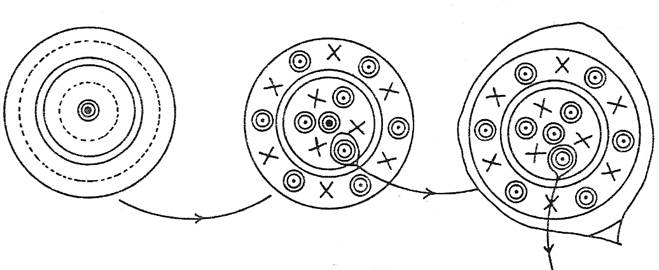●1963:Arnold, V.I. Proof of a theorem by A. N .Kolmogorov on the invariance of quasi-periodic motions under small perturbations of the Hamiltonian. - Usp. Mat. Nauk. SSSR 18, no. 5 Moser's Twist Theorem and Arnold's proof form what is now known as the KAM theorem named after the three authors. Both authors give credit to Kolmogorov for laying the foundation for one of the most important results in stability theory. As mentioned above, Arnold assumed a real analytic function and Moser assumed a smooth function. Because of the Iron Curtain, there was very limited exchange of information. In the end, the Russians though Moser's approach was superior (because real analytic functions are a proper subset of smooth functions), but Moser felt that Kolmogorov's insights were the key element in the KAM Theorem.  Moser also acknowledged the ground-breaking work of Carl Siegel. (However if the four contributors were listed in chronological order, the KAM theorem would be known as the SKAM Theorem.)   In his 1972 text Stable and Random Motions in Dynamical systems Moser looks at the KAM stability issue from the standpoint of smooth functions as well as analytic functions. He also discusses the small divisor problem in the context of complex analytic functions and presents a simplified version of Siegel's proof.   On the left below is a rational torus of the type which can lead to resonances and stability problems. This torus shows a 5:2 resonance between the two windings.  This type of resonance exists between Saturn and Jupiter. If ωJ and ωS are the orbital frequencies of Jupite and Saturn, then 2ωJ - 5ωS ~ 0 and 'small divisor' terms of this type occur in the Fourier series expansion of the motion. (Moser points out that Uranus and Neptune have a ratio which is almost 2 and there is also an interesting relationship between Jupiter, Mars and Earth: 3ωJ - 8ωM +  4ωE ~ 0.)   On the right is the Standard Map which is often used to illustrate the breakdown of tori as perturbations increase and the KAM theorem no longer applies.The perturbation factor shown here is quite large. The magenta orbit is the 'last' invariant torus to survive because it has a winding number equal to the Golden Mean and is therefore poorly approximated by rationals.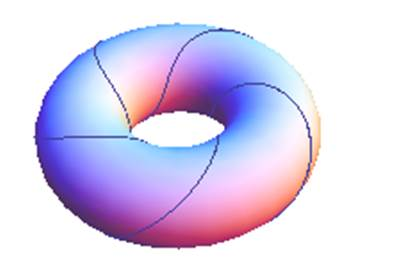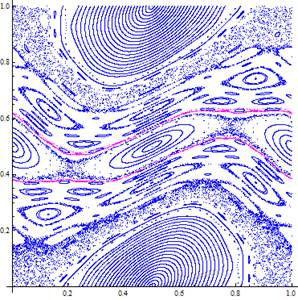●1971:C. L Siegel, J.K Moser . Lectures on Celestial Mechanics, Springer-Verlag, Berlin   In 1952, Jurgen Moser had just received a doctoral degree at Gottingen under Franz Rellich. He attended a lecture series on Celestial Mechanics taught by Carl Siegel who had just returned from Princeton. Moser was encouraged by Rellich to write-up the notes from that lecture series. This was the beginning of a long-term collaboration between Moser and Siegel. The lecture  notes were published in German in 1955 and translated into English in 1971- at which time Moser (now at the Courant Institute in New York) added new material to the chapter on stability theory, to include existence theorems for quasi-periodic solutions which formed the basis of the KAM theorem.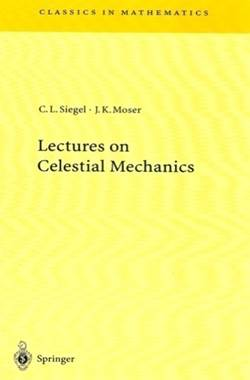●1973: J. Moser, Stable and Random Motions in Dynamical systems, Ann. of Math. Studies, 77, Princeton Univ. Press, Princeton, NJ,  MR0442980 (56:1355)   This was based on a series of five Hermann Weyl lectures given at the Institute for Advanced Study at Princeton in 1972. It includes extensive historical and mathematical background behind the Twist Theorem and the KAM theorem. The Tangent Map illustration below is from the Introduction. He states "As a consequence of Theorem 2.11 (the Twist Theorem) below one can show that every orbit is indeed bounded ifis six times continuously differentiable (C6)" Moser notes that the 'small-divisor' issue arises even in this simple scenario. The full proof of this boundedness was given by Douady in 1982. In his original 1962 proof, Moser needed 333 continuous derivatives (C333) but this was later refined by Russman to a C5 curve and Moser conjectured that it even may hold for C2. Counterexamples exist for the C1 case (F,Takens -1971). Circles and ellipses have no limitations on derivatives so they are C∞. In these cases the orbits are circles or ellipses. For the general C6 case, the orbits are bounded but may not lie on a smooth curve. The C2+epsilon conjecture is still open. Moser makes no mention of the polygonal case in this work.●1978: J. K. Moser  Is the Solar System Stable ?  The Mathematical Intelligencer, Vol. 1, No. 2: 65-71   This was the first 'popular-press' publication about the KAM Theorem and it appeared in the inaugural issue of the Mathematical Intelligencer which is devoted to mathematics and its historical development. It started as a pamphlet in 1971. The cover illustration  is the 'smooth curve' version, but Moser also presents the polygonal version as an example where the KAM theory breaks down. He attributes the Outer-Billiards Map 7 to B.H. Neumann in 1959 but also mentions P.C. Hammer's 1963 work in convexity. Over the next 20 years S. Aubry and J. Mather developed a theory to describe what happens when the invariant KAM curves break down. This is now known as Aubry-Mather Theory and it is concerned primarily with monotonic twist maps and the corresponding closed invariant Cantor sets. (The set of KAM curves has finite measure but they form a Cantor set with no interior so it is impossible to pick an initial condition and say whether it is stable or not.)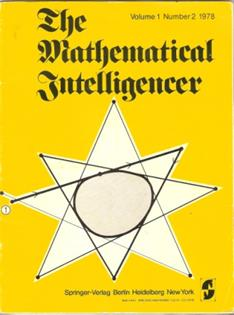●1987 F. Vivaldi, A. Shaidenko, Global stability of a class of discontinuous dual billiards. Comm. Math. Phys. 110 , 625–640. MR895220 (89c:58067)   The authors show that regular polygons and lattice polygons must have bounded orbits under 7 because there are endless rings of 'necklaces' such as the one shown below. The region between the rings is invariant, so orbits are trapped. These rings mimic the quasi-periodic orbits of the continuous case. For regular n-gons, the necklaces are rings of 2n-gon 'Dads' as shown on the right for the regular haptagon. In the case of lattice polygons, the fact that orbits are bounded implies that they are periodic. This is not true for polygons in general and in fact the regular pentagon has orbits which are bounded but not periodic.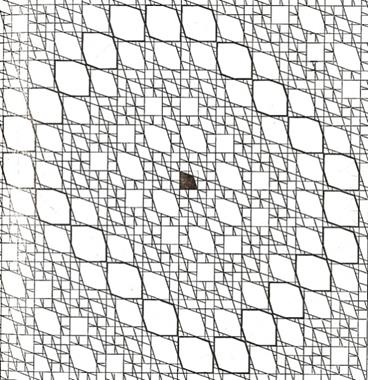●1988 Chua L.O.,Lin T. Chaos in digital filters. IEEE Transactions on Circuits and Systems 35:648-658   In 1968, J.B. Jackson and his colleagues at Bell Labs were trying to understand the source of self-sustaining oscillations in digital filters. Scientists were just becoming aware of the possibility of chaotic behavior in feed-back networks. One of the pioneers in electrical circuit chaos was L.O. Chua at Berkley. He showed that certain digital filter circuits had the potential for chaotic behavior. Some of the discrete mappings used to describe these filters show a remarkable ability to 'shadow' the outer-billiards map when the polygon in question is regular. This connection is discussed in Outer Billiards, Digital Filters and Kicked Hamiltonians at http://arxiv.org/abs/1206.5223.●1995 Tabachnikov, S. On the dual billiard problem. Adv. Math. 115 (1995), no. 2, 221–249. MR1354670   One of the pioneers in the study of outer billiards (and inner billiards) is Serge Tabachnikov. In 1995 he showed that the regular pentagon has a non-periodic orbit which is dense in the inner star region. The web for this region can be generated using the orbit of this point. The web is fractal with dimension Ln/Ln[1/GenScale] ≈  1.241. The first 50,000 points in this non-periodic orbit are shown below.  The pentagon N = 5 is also known as M. It is shown here in 'standard position' with vertex 1 at {0,1}. The coordinates of a non-periodic point with dense orbit are: s = {M][], M][]} - where M[] [] is the first coordinate of vertex 5 of M. The magenta arrow extends from M[] to s.   Since s is on a forward extended edge of M, it cannot have a periodic orbit because it has no inverse image. So points on a forward edge either map to a trailing edge or they are non-periodic. Any point that maps to a trailing edge must have been a point of discontinuity of the outer-billiards map. All discontinuities of 7 for a regular polygon can be uncovered by mapping the forward edges. This is called the (forward) web and it is the closure of the plot below. Mapping the trailing edges under 7-1 will yield the same web in the limit. This is called the inverse web. These webs are only fractal for N = 5, 8, 10 and 12.  Any twice-odd pair such as N = 10 and N = 5 will have congruent webs. It is no coincidence that all of these N-gons have Phi[N] = 2 where Phi is the Euler totient function – so they are classified as ‘quadratic’ polygons.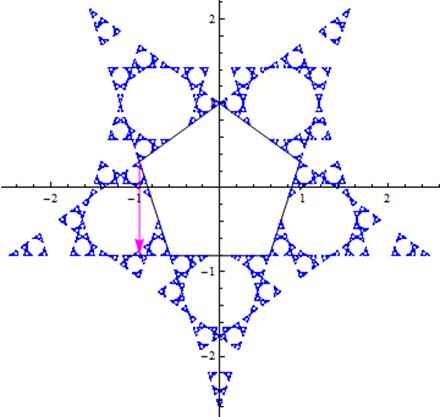●1995 Davies A.C. Nonlinear oscillations and chaos from digital filter overflow. Philosophical Transactions of the Royal Society of London Series A- Mathematical Physical and Engineering Sciences 353:85-99   A.C. Davies and others used the mathematical models of digital filter circuits devised by Chua & Lin in 1988 to develop a dynamical theory based on 'mod-2' mappings of the unit square to itself. These 'torus' mappings (shown in magenta below) yield elliptical centers that mimic the perturbed fixed points of a Hamiltonian system. The elliptical centers can be rectified to circles as shown in the blue rhombus. For certain parameters, the rhombus is an exact copy of the local web for the outer-billiards map. This provides unique approach toward analyzing the dynamics of regular 2n-gons. When n is odd, the web of the n-gon is a subset of the 2n-gon web. Below is the case of N = 14.●1997:Ashwin P, Elliptical behavior in the sawtooth standard map, Physics Letters A 232:409-416   The Chirikov-Taylor Standard Map was devised in the early 1970's. It has become a paradigm for Hamiltonian chaos in the sense that it is an area preserving map with divided phase space where  'integrable' islands of stability are surround by a chaotic component. There have been a number of 'nonsmooth' versions of the Standard Map. P.Ashwin devised a version using a sawtooth non-linearity which he showed is equivalent to the digital filter map used by A.C. Davis. Below is the sawtooth standard map in magenta and the equivalent digital filter map in blue. This provides a connection between the Standard Map and the outer-billiards map. See●2007: R. Schwartz, Unbounded orbits for outer billiards. I. J. Mod. Dyn. 1 , 371–424.MR2318496 (2008f:37082)   When J. Moser discussed the polygonal version of the outer billiards map in 1978, he used it as a model of what might happen when the KAM theorem broke down, so the major issue was "Are there unbounded orbits?". The became known as the Moser-Neumann question. In a sense it is the discrete analog to the question of the stability of the solar system - because these are the issues addressed by the KAM Theorem. In 2007, R.E. Schwartz discovered a class of unbounded orbits for a convex quadrilateral known as a Penrose Kite.   In his more recent 2009 monograph Outer billiards on kites , Richard Schwartz shows that the Tangent Map has unbounded orbits on any irrational kite - that is any kite with a single irrational vertex. He conjectures that this is 'generic' for large classes of polygons. On the left below is a point p which was shown to be non-periodic. The numbers given are the periods of some major 'resonances'. The orbit of p lies entirely on a 1-dimensional lattice composed of lines of the form y = k where k is an odd integer. The illustration on the right is a colored version of this same web, used in the Math Book by Clifford Pickover.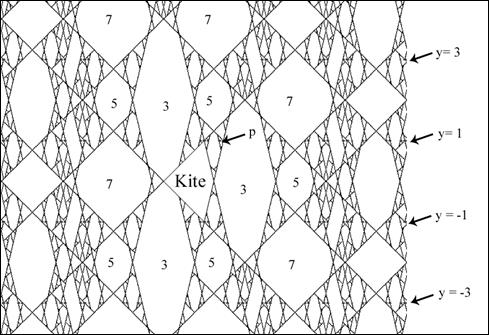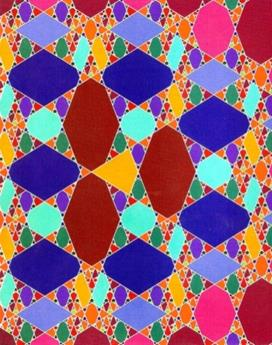●2008: . D. Genin, Research announcement: boundedness of orbits for trapezoidal outer billiards. Electronic Research Announc. Math. Sci. 15 , 71–78. MR2457051 (2009k:37036)   The mathematical definition of a trapezoid is a quadrilateral with at least two sides parallel, so the parallelograms are special cases of trapezoids. There is no affine transformation that would turn a trapezoid into a kite. When a trapezoid is a lattice, it is known that all orbits are bounded, but D. Genin proved that this is true for all trapezoids. The web for an arbitrary trapezoid is shown below.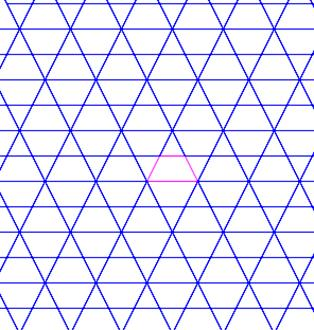●2009. D. Dolgopyat, B. Fayad, Unbounded orbits for semicircular outer billiard. Ann. Henri Poincare 10  357–375. MR2511890 (2010d:37076)   The Moser-Neumenn question was directed at both smooth (differentiable) curves and polygons. For smooth curves, the only known integrable cases are the circle and the ellipse. In both cases the Tangent Map preserves circle and ellipses, so these are integrals of motion. This is true for inner billiards as well. For inner billiards, George Birkhoff conjectured that these are the only two integrable cases. This has not been proven, but it may hold for both inner and outer billiards.   Moser and Douady used the Twist Theorem to show that if the generating curve is C6 (six times continuously differentiable), then all orbits are bounded.A semi-circle has elements of both the smooth and non-smooth curves. Like a polygon, it is C0 (continuous) but not C1 (differentiable). In 2009  D. Dolgopyat and B. Fayad showed that a semi circle has unbounded orbits that diverge in spiral patterns. Below is an approximation to a semi circle using a truncated regular polygon with 200 sides. The magenta orbit on the right, started in close proximity to the semi-circle - which is just a speck on this scale.This orbit shows typical divergent behavior.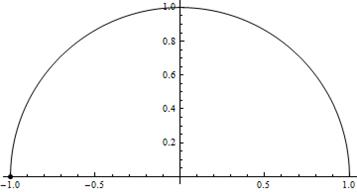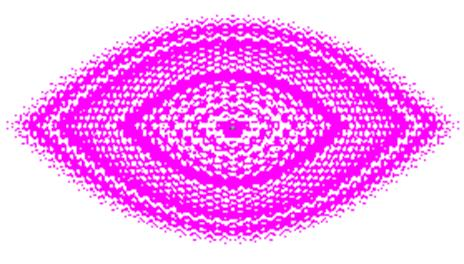●2010 R. Schwartz, Outer Billiards, Arithmetic Graphs, and the Octagon. arXiv:1006.2782.   The cases of N = 5, 8 , 10 and 12 are the only known cases where the web structure is a perfect fractal. In all these cases it appears that a single non-periodic orbit is dense in the web -but this has not been proven for N = 12. As described above the fractal dimension of the regular pentagon is Ln[5+1]/Ln[1/GenScale] ≈   1.241 and it is not hard to show that the fractal dimension of the regular octagon is Ln[8+1]/Ln[1/GenScale] ≈ 1.246.   There is only one non-redundant way to 'remap' the vertices of a regular octagon: {1,2,3,4,5,6,7,8} →{1,4,7,2,5,8,3,6}.This mod-3 remapping of the non-periodic orbit is what generates the fractal Koch snowflake on the right below. The mod-2 remapping is redundant but it generates the fractal 'sponge' in the middle, and the mod-1 'remapping ' is the original (return) orbit which is shown on the left below.  These are called 'projections' since they map R8→R2. They are also examples of the arithmetic graphs that Richard Schwartz used to prove that the Penrose kite had unbounded orbits.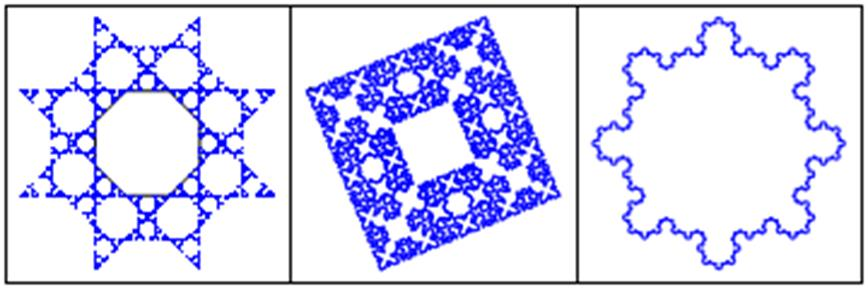●2012 G.H. Hughes, Outer Billiards on Regular Polygons - arxiv.org/abs/1311.6763   In 1989 F.Vivaldi and A. Shaidanko showed that all outer-billiards obits of a regular polygon are bounded and in that same year the author met with J. Moser at Stanford University to discuss the ‘canonical’ structures that always arise in the singularity set W when N is regular. Moser suggested that a study of these structures would be an interesting exercise in ‘recreational’ mathematics. This is exactly what it became over the years - but the evolution of these canonical ‘First Families’ proved to be a difficult issue except for regular N-gons with linear or quadratic complexity. This paper introduces these canonical First Families and their connection with the outer-billiards map.   Below is the First Family of N = 14. Note that the odd S[k] and DS[k] have two distinct forms as magenta 14-gons and cyan 7-gons. Such S[k] are called ‘androgynous’ because even and odd N-gons have different dynamics and the hence different ‘parity’ or ‘gender’. Only twice-odd N-gons can support both genders in their First Families. By the Twice-odd Lemma, there is an invertible transformation T that would map this First Family of N = 14 to the First Family for N = 7. In this transformation, T[S] would be N = 7 so the cyan S here can be regarded as a scaled copy of N = 7. This is true because N = 14 and N = 7 have equivalent cyclotomic fields and all the algebraic structure of a regular N-gon is contained in the cyclotomic field QN. As a vector space over the rationals Q, the cyclotomic field is generated by the (complex) vertices of N. The sequel article below has recently been updated. It shows First Family scales can be used to generate the maximal real subfield of QN which is denoted QN+. This is called the scaling field of N.●2019 G.H. Hughes, Families of Regular Polygons and their Mutations - arxiv.org/abs/1612.09295   As illstrated above, every regular N-gon generates a canonical ‘family’ of regular polygons which are conforming to the bounds of the ‘star polygons’ determined by N. These star polygons are formed from truncated extended edges of the N-gon and the intersection points (‘star points’ shown above in blue) determine a scaling which defines the parameters of the family. Based on a 1949 result of Carl Siegel it follows that this star-point scaling forms a unit basis for the maximal real subfield of the cyclotomic field QN. The traditional generator for this subfield is 2cos(2π/N) so it has order Phi(N)/2 where Phi is the Euler totient function. This order is known as the ‘algebraic complexity’ of N. The family of conforming regular polygons shares the same scaling and complexity as N, so it is called the First Family of N.   This scenario is illustrated in steps (i) and (ii) here for N = 14. (The cyan First Family shown here is identical to the illustration above.) This is independent of any mapping but for piecewise ‘rational’ isometries such as the outer-billiards map tau, the digital filter map Df , or the dual-center map Dc, the singularity set W can be formed by iterating these extended edges of N under tau , Df or Dc. This is illustrated in (iii) below. This singularity set W is generated by the outer-billiards map tau but Df and Dc will generate congruent versions of W. This is also called a ‘generalized star polygon’ since it is generated by the edges of the star polygon of N. It retains the same dihedral symmetry group as N.Section 4 shows that W preserves the S[k] and Lemma 4.1 says that tau-orbit of each S[k] has a constant step-k periodic orbit. Therefore the S[k] mimic the classical period-based ‘resonances’ from Hamiltonian dynamics and the Standard Map. (See the illustration below). In addition the ‘web’ W can be regarded as the disjoint union (coproduct) of the local webs of the S[k]. These local webs are still very complex but for N-gons with complexity that is linear or quadratic (N = 3,4,5,6,8,10,12), this S[k] evolution is sufficient to describe the resulting topology of W - because in these cases there is never more than one effective scale. Therefore the topology is linear for N = 3, 4 and 6 and simple fractal for N = 5,8,10,12.      When there are multiple non-trivial scaling parameters, the topology of W may be multi-fractal and there is no guarantee that the First Family scaling will be sufficient to determine the geometric scaling of W - but the Scaling Conjecture says that this scaling in QN+ is always sufficient.   Below is a connection between the Standard Map – which simulates the phase-space dynamics of the KAM Theorem - and the digital filter map Df – which generates a web that is conjugate to W.  At the bottom we show the connection with the complex-valued dual-center map Dc. This map also generates a web which is conjugate to W. These connections are described in Outer Billiards, Digital Filters and Kicked Hamiltonians.This last image shows a remarkable chain of equivalences that begins with the Standard Map – because P. Ashwin devised a ‘sawtooth’ version of the Standard map which allows it to be ‘tuned’ to N = 14  (shown here in magenta) . Under a simple change of variables this sawtooth map is equivalent to the digital-filter map (in blue above) and with the right parameters the digital-filter map is conjugate to the outer-billiards web W for N = 14 (in black).   Since the Standard Map is just a graphical representation of the phase space for the KAM Theorem, this chain shows a direct connection between the ‘resonant’ islands of stability of the KAM Theory and the S[k] of the First Family. * Thanks to John Mather of Princeton University for his insights into the mathematics and politics of the KAM Theorem.